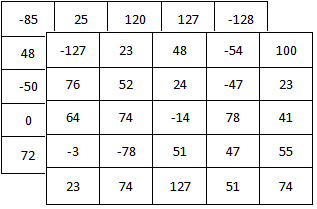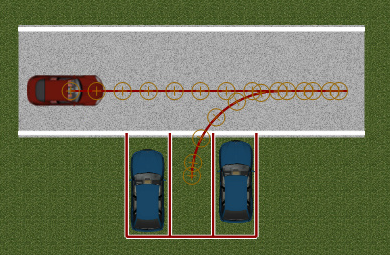• 先贴上我总结的Opencv的数据类型, 主要是针对不同Mat类型进行新建,修改和访问时使用, 更详细的数据访问见下文: 2. CV_8UC3解说 新建一个CV_8UC3型的cv::Mat, 其中U代表了unsigned char型的数据, 其表示的范围为0 到...

1. 总结

先贴上我总结的Opencv的数据类型, 主要是针对不同Mat类型进行新建,修改和访问时使用, 更详细的数据访问见下文:2. CV_8UC3解说

新建一个CV_8UC3型的cv::Mat, 其中U代表了unsigned char型的数据, 其表示的范围为0 到 255; C3表示三个通道, 也就是同一个位置能存放几个数字;
最常见的使用位置是用于保存图像类型, C3用于存储RGB彩色图像, C1用于存储灰度图像, 为什么用uchar型数据来存储图像数据, 这里有详细的解释.
总结来讲,就是int型的数据表示范围过大, 如果使用int型, 一副图像所占内存有点大, 而 uchar型数据范围刚好用来保存256级的像素, 节省空间;
具体的访问:

// CV_8UC3 的数据类型集访问
std::string image_path = myRGBimagePath;
std::cout << "input_image type: "<< input_image.type() << std::endl; //CV_8UC3 16
// 对uchar数据的访问, 三通道, 数据为uchar型, 显示时要用(int)进行强制转换
cv::Vec3b a_pixel = input_image.at<cv::Vec3b>(0,0); //127, 130, 128
//三个数字位于相同的像素位置, 但是属于不同的通道
std::cout << "pixel in center: " << (int)a_pixel << ", "<<  (int)a_pixel << ", " <<  (int)a_pixel << std::endl;
//3. 8UC3转换成8UC1, 访问时直接使用uchar
cv::Mat input_gray(input_image.size(), CV_8UC1);
cv::cvtColor(input_image,input_gray,CV_RGB2GRAY);
std::cout << "input_gray <0,0> " << (int)input_gray.at<uchar>(0,0) <<std::endl;

3. CV_8SC2详解

接下来说一下不常见的CV_8S类型的数据, 以CV_8SC2为例, S代表了有符号 signed char, 8就代表了这个数据可以用8bit表示, 上面的unsigned char 型, 8bits 表示范围是0~255, 那么这里的signed char型表示的范围自然就正负各一半: - 128 到 127; C2代表了两个通道; 下面举个例子:其中的每个元素都是8位的有符号整型;
假如这是5*5的Mat, 那么(0,0) 位置上有两个元素存放, 为(-85, -127);

这里对signed char型多通道Mat的访问, 如何用at实现,我还不太清楚, 这也就是为什么上面表格中第二行没有对应的Vec, 有了解的欢迎不吝赐教啊!!

这里举一个用行指针实现的Mat的访问:

//不同的数据类型的Mat可以通过convertTo() 函数进行转换
cv::Mat input_image_schar;
input_image.convertTo(input_image_schar, CV_8SC3);
//先读取第0行的数据
schar* mypixel = input_image_schar.ptr<schar>(0);
std::cout << "mypixel = " << (int)mypixel << "," <<(int)mypixel << ","<<(int)mypixel << "," << std::endl;

有更多基础知识想要了解的,可以看这个OpenCV Tutorial (查看图片可能需要翻墙)

4. InputArray 与cv::Mat

cv::InputArray() 是opencv建立的一个更包容的类, 一般用在OpenCV的函数接口处; 可以承接Mat、Mat_、Mat_<T, m, n>、vector <T>、vector<vector<T>、vector<Mat> 类型的数据; 这里简单地与Mat做一个转换:

std::vector<cv::Point2f> myPoints_xy;
myPoints_xy.push_back(cv::Point2f(2.45,3.7));
myPoints_xy.push_back(cv::Point2f(3.68,8.8));
myPoints_xy.push_back(cv::Point2f(8.55,8.12));
//可以利用 vector<cv::Point2f>来初始化一个InputArray
cv::InputArray myArray_xy(myPoints_xy);
std::cout << "myArray_xy type: " << myArray_xy.type() << "myArray_xy size: " << myArray_xy.size()<< std::endl;
cv::Mat myMat_xy;//获取到矩阵数据并转换成CV_32FC2的类型
myArray_xy.getMat().convertTo(myMat_xy,CV_32FC2);
//CV_32F数据类型的访问也是比较简单易懂, 直接找到对应的Vec即可
cv::Vec2f tmpPoint = myMat_xy.at<cv::Vec2f>(0,0);
std::cout << "tmpPoint is " << tmpPoint << "," << tmpPoint << std::endl;//

output:
myArray_xy.type() = 13: CV_32FC2 size: [3 x 1]
tmpPoint is 2.45,3.7

5. Mat的不同访问方式

int main()
{
cv::Mat matF(3, 3, CV_32F);
randu(matF, Scalar(0), Scalar(10));

int rowIdx = 1;
int colIdx = 1;

// 1. 直接使用at<数据类型>(行值,列值)来访问
float f1 = matF.at<float>(rowIdx, colIdx);

// 2. 使用.data 获取数据存储区域的第一个数据的位置, step1() 代表的是一行总共有几个数据,3x3的CV_32FC4 一行有4*3=12个数据
float* fData2 = (float*)matF.data;
float f2 = fData2[rowIdx*matF.step1() + colIdx];

// 3. 使用.ptr<数据类型>(0) 获取第一个元素的地址
float* fData3 = matF.ptr<float>(0);
float f3 = fData3[rowIdx*matF.step1() + colIdx];

// 4.使用.ptr<数据类型>(行数) 的行指针形式进行访问
float* fData4 = matF.ptr<float>(rowIdx);
float f4 = fData4[colIdx];

// 5. typedef Mat_<float> Mat1f, 数据访问会更加快速
Mat1f mm(matF); // Or directly create like: Mat1f mm(3, 3);
float f5 = mm(rowIdx, colIdx);

// f1 == f2 == f3 == f4 == f5

return 0;
}

这部分代码参考:　SO上的问题
对于Mat的访问想进一步了解的, 可以参考我的这篇小白学习OpenCV Mat, 可能对于Step, Channel那些会有进一步的了解.

有什么问题,欢迎大家多多交流~~

展开全文opencv c++ Mat uchar 数据类型
• 1.npy格式 1.1 读取 读取npy格式文件 data_a=np.load('C:/Users/YX/Desktop/data.npy') 1.2 储存 import numpy as np a = np.array([1,2,3],[4,5,6]) np.save('adress/name',a) ...2.mat格式（暂未更新，有空会来记录

1.npy格式

1.1 读取

读取npy格式文件

import numpy as np

1.2 储存

import numpy as np
a = np.array([1,2,3],[4,5,6])

name：存储的名称
例如：C:/Users/YX/Desktop/data
最后就会在桌面上有一个名称为data的npy格式文件

2.mat格式

1.1 读取

import scipy.io as scio

说明：-------------------------------↑1-----------------------------------↑2-----↑3—
箭头1为：数据存储的位置
箭头2为：数据在matlab中的名称
箭头3为：你需要数据中的哪一行

1.2 储存

# 储存mat
import scipy.io as scio
datafile = 'C:/Users/YX/Desktop/Zxx.mat'
scio.savemat(datafile,{'data':Zxx_new})

说明：
第一行中：datafile为存储的文件地址，最后的Zxx.mat为最终存储的文件名
第二行中：data为数据导入matlab后，matlab里显示的名称
Zxx_new为python中变量的名称

展开全文python 开发语言 后端
• mat文件(mat文件怎么查看)

千次阅读 2021-04-24 01:37:48
另外请说明一下m文件、mat文件、mex文件的概念及异同mat数据格式是matlab的数据存储的标准格式。你可以调用matlab的子程序库，用c或fortan调用mat格式的数据。我给你几个函数名matopen 打开mat文件matclose 关闭mat....

另外请说明一下m文件、mat文件、mex文件的概念及异同

mat数据格式是matlab的数据存储的标准格式。你可以调用matlab的子程序库，用c或fortan调用mat格式的数据。我给你几个函数名matopen 打开mat文件matclose 关闭mat.

谢谢 各位了 已经 回来 谢谢

mat文件. 如果你的计算机装了office Access 那么 它的默认打开很有可能被更改成Access 手动更改默认打开方式也无效. 同时它的扩展名也被隐藏 更改浏览器，让其显示扩.

生成MAT文件 假如你的矩阵A.B都已赋值，现在需要将A,B矩阵保存成mat格式文件，执行如下命令：save mydata A B 就会把A B矩阵数据保存在mydata.dat文件中了 保存.

mat数据格式是matlab的数据存储的标准格式。 你可以调用matlab的子程序库，用c或fortan调用mat格式的数据。 我给你几个函数名 matopen 打开mat文件 matclose 关闭.

在MATLAB中.mat文件有两种读取方法：1. 从MATLAB工作目录下找到该文件，双击；2. 使用MATLAB函数。下面举例说明如何使用MATLAB函数读取和调用.mat文件：% .

mat是存储在workspace中。它是表示对应变量的存储数据。对应变量D的数据

＂.fig＂简单的说就是figure文件用来储存图形界面的，而“.mat”则是用来存储一些变量的文件根word和excel类似。两者没有什么必然关联.

生成mat文件假如你的矩阵a.b都已赋值，现在需要将a,b矩阵保存成mat格式文件，执行如下命令：save mydata a b 就会把a b矩阵数据保存在mydata.dat文件中了保存指定.

在matlab中.mat文件有两种读取方法：1. 从matlab工作目录下找到该文件，双击；2. 使用matlab函数。下面举例说明如何使用matlab函数读取和调用.mat文件：% 假设data..

在matlab中把txt文件保存为.mat的方法：1、用matlab打需要保存的TXT文件。2、选择文件菜单中的另存为。3、在弹出的对话框中，选择保存类型为(*.mat)。4、如果保.

首先你画图之前要有数据 比如说plot(a) 那么你只能保存a 即你的workspace里面要有. 然后在workspace里面保存 在file里面然后就有save workspace as 就是.mat格式了

打开需要转换的文本文档，选择文件——另存为，： 1，选择文件类型为“所有文件”。2、将2处标记的txt改为dat即可。

打开需要转换的文本文档，选择文件——另存为，： 1，选择文件类型为“所有文件”。2、将2处标记的txt改为dat即可。

Matlab写程序的文件。MATLAB是解释型语言，就是说MATLAB命令行中敲入的命令在当前MATLAB进程 中被解释运行。但是，每次执行一个任务时敲入长长的命令序列是.

matlab中读取mat文件和dat文件，dat文件运行速度更快。Matlab MAT-文件 mat数据格式是matlab的数据存储的标准格式。mat文件是标准的二进制文件，还可以ASCII码形.

展开全文• 轨迹数据保存在Trajectories文件夹下，保存为Trajectory_1.mat。 打开Trajectory_1.mat数据维度为25*186。行数固定为25，列数与轨迹长度相关。本例中仿真步长为0.01s，轨迹共运行186步。 前18行中，每行对应的...

环境：PreScan 8.5.0

绘制一条图示轨迹，名为Trajectory_1。轨迹数据保存在Trajectories文件夹下，保存为Trajectory_1.mat。打开Trajectory_1.mat，数据维度为25*186。行数固定为25，列数与轨迹长度相关。本例中仿真步长为0.01s，轨迹共运行186步。

前18行中，每行对应的参数含义如下所示。字母x,y,z表示三种坐标，字母R,P,Y表示三种角度，字母v表示速度，字母a表示加速度。坐标为轨迹点在起始位置的车辆坐标系下的值，而不是全局坐标系下。角度为车辆坐标系与全局坐标系的夹角。

1,2,3 --- x,y,z

4,5,6 --- R,P,Y

7,8,9 --- vx,xy,xz

10,11,12 --- vR,vP,vY

13,14,15 --- ax,ay,az

16,17,18 --- aR,aP,aY

注：

Regenerate后，Trajectory_1.mat不受影响。但是在GUI界面中重新编译后，修改过的Trajectory_1.mat将会被还原为初始状态。

展开全文• 满意答案janey184推荐于 2018.05.14采纳率：58%等级：9已帮助：2315人假如你的矩阵A.B都已赋值，现在需要将A,B矩阵保存成mat格式文件，执行如下命令：save mydata A B 就会把A B矩阵数据保存在mydata.dat文件中了...
• MATLAB存储mat文件，数据大小超过2GB，采用-v7.3存储; 不超过的话采用一般格式 在Python中读取MATLAB的.mat文件一般使用scipy，但是scipy不支持-v7.3版本的.mat文件（-v7.3版本能支持较大的文件）；因此使用h5py...python matlab
• MNIST手写数字图像数据库60000个训练集，10000个测试集，灰度图，大小均为28*28数据集网址：http://yann.lecun.com/exdb/mnist/train-images-idx3-ubyte.gz: training set images (9912422 bytes)train-labels-idx1-...
• MATLAB利用save命令将数据保存为mat格式matlab
• 实验所使用的数据集作为实验的重要组成部分，我们往往需要对拿来的数据集进行数据预处理工作，因为通常使用的数据集往往都是来自各行各业的数据，而一个数据集往往具有多个属性，每个属性的值是否使用的同一量纲，这...python 数据集 归一化
• 以http://ufldl.stanford.edu/housenumbers/上的mat数据集为例需要注意以下几点从mat提取出来的数据以字典的形式保存，所以需要提取字典的key和valueimport numpy as npimport osfrom PIL import Imageimport ...
• 满意答案janey184推荐于 2018.05.14采纳率：58%等级：9已帮助：2315人假如你的矩阵A.B都已赋值，现在需要将A,B矩阵保存成mat格式文件，执行如下命令：save mydata A B 就会把A B矩阵数据保存在mydata.dat文件中了...
• mat = scipy.io.loadmat('{}/cls/{}.mat'.format(self.sbdd_dir, idx)) mat = mat['GTcls']['Boundaries'] gt_ = np.empty((mat.shape, in_.shape, in_.shape), dtype=np.uint8) for i in range...
• 在Python中处理MATLAB的*.mat格式数据及常见错误汇总前言一、数据读取错误二、数据类型错误（用Python处理图像时，若涉及加减运算，溢出差值被重新赋值255-0） 欢迎学习交流！ 个人网站：...python matlab 编程语言
•matlab python
• #include #include using namespace cv;using namespace std;... //控制台中文支持Mat r = Mat(10, 3, CV_8UC3);randu(r, Scalar::all(0), Scalar::all(255)); //randu随机矩阵[0, 255]之间cout &...
• import scipy.io as sio mat = sio.loadmat('D:\大创比赛\H_4T4R.mat') data = mat['H_4T4R']
• 前言Mat(图像数据结构体)代替了CvMat和IPIImage，并将功能模块化。Mat它由两个数据部分组成：矩阵头(包含矩阵尺寸，存储方法，存储地址等信息)和一个指向存储所有像素值的矩阵(根据所选存储方法的不同矩阵可以是不同...
• 突然发现有一篇opencv操作mat数据的基础操作搞忘了，现在发出来，原来有一篇是只有操作结果,而且是用emgu写的，这里用c++实现并给出详细的代码。数字图像处理其实就是处理二维矩阵数据。利用opencv来学习处理算法是...c++ mat赋值
• mat格式转换

2021-04-21 11:32:16
1. mat文件转txt文件 .mat 是matlab专用的文件，可以先把文件读入matlab，再用 fprintf 或者... 比如 %把数据加载进matlab，heart_scale.mat 有两项：heart_scale_init 和 heart_scale_lmatlab怎么将mat文件转换成tx...
• 应用：将三通道彩色图的Mat数据，转存为单通道灰度图的unsigned char*数据：Mat ImageMedia; //读取unsigned char *pGrayImage;//存入int xsize = ImageMedia.cols;int ysize = ImageMedia.rows;pGrayImage = new ...
• data：Mat对象中的一个指针，指向内存中存放矩阵数据的一块内存 (uchar* data)dims：Mat所代表的矩阵的维度，如 3 * 4 的矩阵为 2 维， 3 * 4 * 5 的为3维channels：通道，矩阵中的每一个矩阵元素拥有的值的个数，...
• 这两天在搞Theano，要把mat文件转成pickle格式载入Python。Matlab是把一维数组当做n*1的矩阵的，但Numpy里还是有vector和matrix的区别，Theano也是对二者做了区分。直接把代码贴出来吧，好像也没什么可讲的 = =from ...
• 尽管OPENCV已经升级了N多次，最新版本是2.4.6，但对数据的访问一直还是延续OPENCV1.X中讲解的效率最高的访问方式，也即指针方式。今天偶来兴致，想测测自己频繁使用访问数组方式的各种效率，结果令人惊讶(本测试是在...
• import scipy.io as sio import skimage.io 读入tif图 imgpath = r'E:\高光谱数据\014140790010_01\014140790010_01_P001_MUL\19AUG26035324-...转为mat sio.savemat(r"E:\高光谱数据\014140790010_01\014140790010_0
• MATLAB提供了多种方式从磁盘读入文件或将数据输入到工作空间，...● 数据格式为文本格式、二进制格式还是如HDF之类的标准格式。将数据导入MATLAB中最容易的方法就是使用导入数据模板(Import Wizard)，使用该模板时...
• filename = r'D:\python_project_lzz\a1405.mat' data = loadmat(filename) dfdata = pd.DataFrame(data=data['data'][1:],columns=['date','time','open','high','low','close','volume','amount','position']).as...
• 该楼层疑似违规已被系统折叠隐藏此楼查看此楼如图我想把每列的数据平方之后相加再开根号，之后用matlab画图，matlab程序应该怎么写我有画这三列图像的M文件%%%%%%%%%%%%%%%%%%%%%%%%%%%%%%%%%%%%%%%%%%%%%%%%%%%%%%%...
• matlab的mat格式

2021-04-23 14:18:19
MAT文件是matlab专用于保存数据至磁盘和想matlab导入、从matlab导出数据数据文件格式MAT提供了一种简单的机制，它允许两个平台之间以灵活的方式移动数据。为了简化在matlab环境之外对MAT文件的使用，matlab给出...
• 前言很多人不重视Mat数据类型，其实，数据类型不对往往会导致溢出、截断等各种问题，使得计算结果看起来不对，让人陷入迷茫。这篇文章我们来深入聊聊Mat数据类型以及他们之间的转换。Mat有的数据类型OpenCV的......